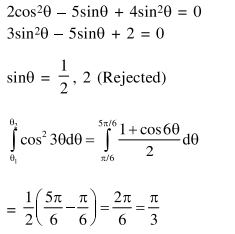Deepak Scored 45->99%ile with Bounce Back Crack Course. You can do it too!

# If theta_1 and theta_2 be respectively the smallest

Question:

If $\theta_{1}$ and $\theta_{2}$ be respectively the smallest and the largest values of $\theta$ in $(0,2 \pi)-\{\pi\}$ which satisfy

the equation, $2 \cot ^{2} \theta-\frac{5}{\sin \theta}+4=0$, then

$\int_{\theta_{1}}^{\theta_{2}} \cos ^{2} 3 \theta d \theta$ is equal to :

1. $\frac{2 \pi}{3}$

2. $\frac{\pi}{3}+\frac{1}{6}$

3. $\frac{\pi}{9}$

4. $\frac{\pi}{3}$

Correct Option: , 4

Solution: